##### Geometry For Dummies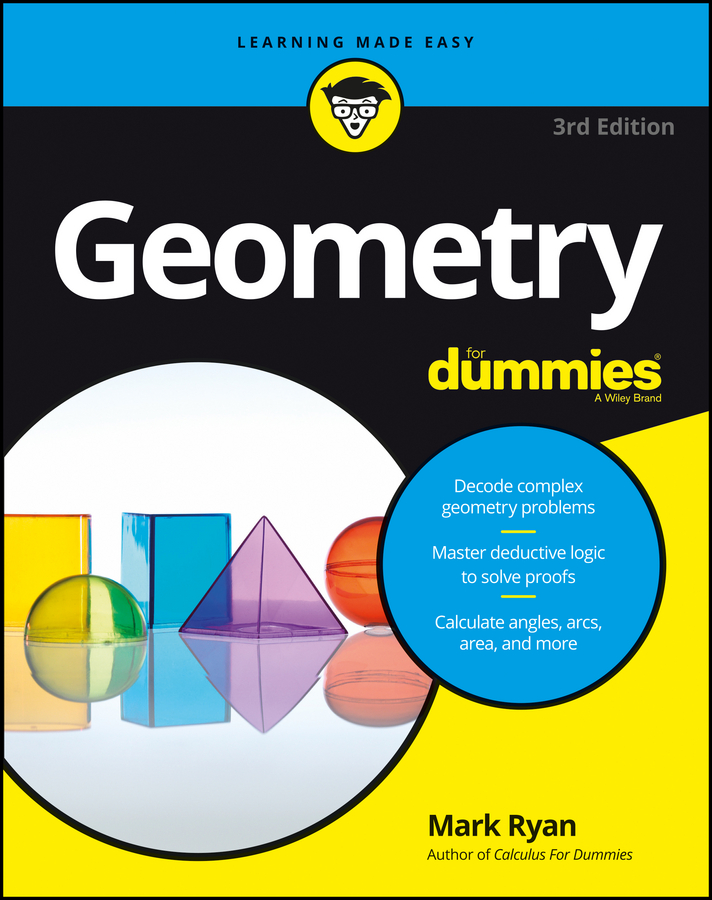Recall that similar polygons are polygons whose corresponding angles are congruent and whose corresponding sides are proportional. The figure below shows similar pentagons, ROTFL and SUBAG.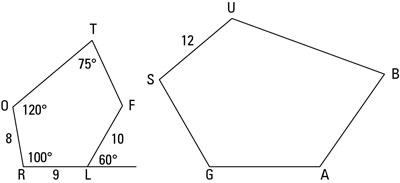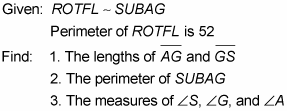You can see that ROTFL and SUBAG aren’t positioned the same way just by looking at the figure (and noting that their first letters, R and S, aren’t in the same place). So you need to figure out how their vertices correspond. Try using one of the methods from the following list:

• You can often tell how the vertices correspond just by looking at the polygons, which is actually a pretty good way of seeing whether one polygon has been flipped over or spun around.

• If the similarity is given to you and written out like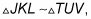you know that the first letters, J and T, correspond, K and U correspond, and L and V correspond. The order of the letters also tells you that segment KL corresponds to segment UV, and so on.

• If you know the measures of the angles or which angles are congruent to which, that information tells you how the vertices correspond because corresponding angles are congruent.

• If you’re given (or you figure out) which sides are proportional, that info tells you how the sides would stack up, and from that you can see how the vertices correspond.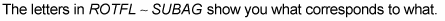R corresponds to S, O corresponds to U, and so on. (By the way, do you see what you’d have to do to line up SUBAG with ROTFL? SUBAG has sort of been tipped over to the right, so you’d have to rotate it counterclockwise a bit and stand it up on base segment GS. You may want to redraw SUBAG like that, which can really help you see how all the parts of the two pentagons correspond.)

1. Find the lengths of segment AG and segment GS.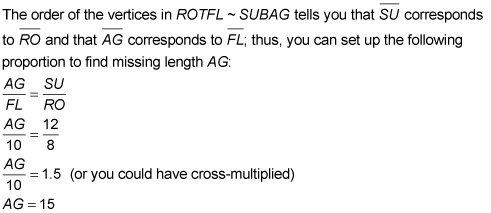This method of setting up a proportion and solving for the unknown length is the standard way of solving this type of problem. It’s often useful, and you should know how to do it (including knowing how to cross-multiply).

But another method can come in handy. Here’s how to use it to find GS:

Divide the lengths of two known sides of the figures like this: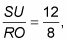which equals 1.5. That answer tells you that all the sides of SUBAG (and its perimeter) are 1.5 times as long as their counterparts in ROTFL. You can think of the number 1.5 as the expansion factor or expansion multiplier that expands ROTFL to the size of SUBAG.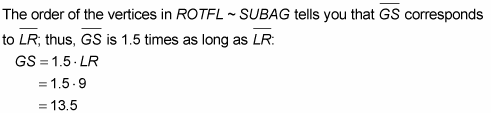2. Find the perimeter of SUBAG.

The alternative method introduced above tells you immediately that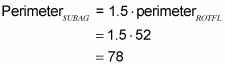But for math teachers and other fans of formality, here’s the standard method using cross-multiplication: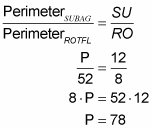3. Find the measures of angles S, G, and A.

S corresponds to R, G corresponds to L, and A corresponds to F, so

• Angle S is the same as angle R, or 100°.

• Angle G is the same as angle RLF, which is 120° (the supplement of the 60° angle).

To get angle A, you first have to find angle F with the sum-of-angles formula: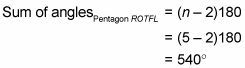Because the other four angles of ROTFL (clockwise from L) add up to 120° + 100° + 120° + 75° = 415°, angle F, and therefore angle A, must equal 540° – 415°, or 125°.